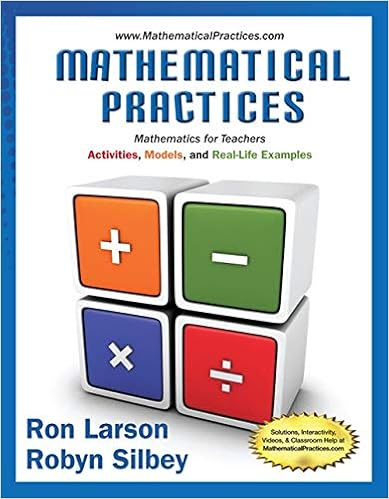26 27 28 select the negation of the following

• Homework Help
• 14
• 100% (4) 4 out of 4 people found this document helpful

This preview shows page 7 - 9 out of 14 pages.

We have textbook solutions for you!
The document you are viewing contains questions related to this textbook.The document you are viewing contains questions related to this textbook.
Chapter 1 / Exercise 17
Mathematical Practices, Mathematics for Teachers: Activities, Models, and Real-Life Examples
LarsonExpert Verified
Select the negation of the following statement. If , then . the sky does not look blue it is night time , and . The sky looks blue it is not night time , and . The sky does not look blue it is not night time If , then . the sky looks blue it is not night time If , then . it is not night time the sky looks blue Problem Set 7 Sections 3.3, 3.4-Dakota Rasimas
27. Write the statement as an equivalent statement that does not use the if...then connective. Remember that is equivalent to p q ~ p q If , then . we go to the movies we buy popcorn Choose the equivalent statement below. .
28. Write the following statement as an equivalent statement that does not use the if-then connective. Remember that p is equivalent to ~p q. If you scratch my back, I'll scratch yours. Choose the correct answer below. q
7 of 14 3/13/18, 9:50 PM
26. Write the negation of the statement. If it is not , then it is . blue lettuce Choose the correct negation below.
We have textbook solutions for you!
The document you are viewing contains questions related to this textbook.The document you are viewing contains questions related to this textbook.
Chapter 1 / Exercise 17
Mathematical Practices, Mathematics for Teachers: Activities, Models, and Real-Life Examples
LarsonExpert Verified
29. Use truth tables to decide whether the pair of statements is equivalent. ; p q ~ q p Are the statements equivalent? A. The statements are not equivalent. When p is true and q is false, the statements have different truth values. B. The statements are not equivalent. the same truth value. When both p and q are true, the statements have C. The statements are equivalent. The statements have the same truth value for all values of p and q. D. The statements are equivalent. When both p and q are false, the statements have different truth values.
30. and ~ p ~ q ~ q ~ Choose the correct answer below. . The statements are not equivalent . The statements are equivalent Use truth tables to decide whether the pair of statements is equivalent. and ~ r ~ s s Are the statements equivalent? 31. Use a truth table to determine whether the two statements are equivalent. and ~ p ~ q ~ q ~ Choose the correct answer below. . The statements are not equivalent . The statements are equivalent Use truth tables to decide whether the pair of statements is equivalent. and ~ r ~ s s Are the statements equivalent? p r
•••